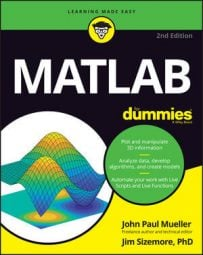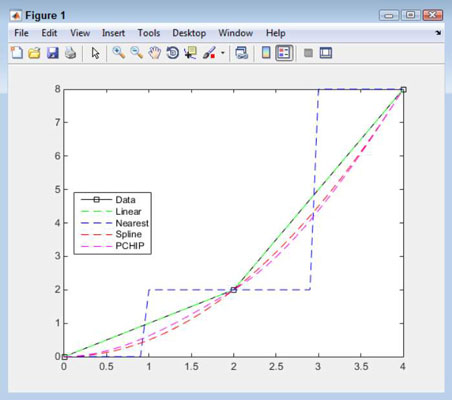##### MATLAB For DummiesMATLAB lets you perform a wide range of algebraic tasks even without the Symbolic Math Toolbox installed, but adding the Toolbox makes performing the tasks easier. Here, you discover how to use the Symbolic Math Toolbox to perform a variety of algebraic tasks.

## Differentiating between numeric and symbolic algebra

The essential difference between numeric and symbolic algebra is that the first is used by computer science to explore principles of algebra using symbols in place of values, while the second is used by science to obtain approximations of equations for real-world use. When you want to perform numeric algebra, you use the vpasolve() function. The following steps demonstrate how to perform this task:

1. Type syms x y and press Enter.

Even when performing numeric algebra, you must define the variables before you use them.

2. Type vpasolve(2 * x + 3 * y - 22 == 0, x) and press Enter.

You see the following output:

```ans =
11.0 - 1.5*y```

The output is simpler this time, but notice that it also relies on floating-point numbers. In order to ensure precision, symbolic algebra relies on integers. A floating-point number is an approximation in the computer — an integer is precise.

When working with vpasolve(), you must specify which variable to solve for. In this case, vpasolve() solves for x.

3. Type vpasolve(11.0 - 1.5*y) and press Enter.

You see the following output:

```ans =
7.3333333333333333333333333333333```

The output is a floating-point number. You aren’t dealing with a fraction any longer, but the number is also an approximation. You need to note that vpasolve() defaults to providing 32-digit output — a double is 16 digits.

4. Type vpasolve(2 * 7.3333333333333333333333333333333 + 3 * y - 22 == 0) and press Enter.

You see the following output:

```ans =
2.4444444444444444444444444444444```

Again, the output is a floating-point number. The result is imprecise. However, seeing whether the computer can actually show you how much it’s off might be interesting.

5. Type 2 * 7.3333333333333333333333333333333 + 3 * 2.4444444444444444444444444444444 - 22 and press Enter.

MATLAB likely outputs a value of 0. The point is that the two output values truly aren’t precise values, but the computer lacks the precision to detect just how much of an error exists.

There are times when using the Symbolic Math Toolbox makes things easier but using it isn’t absolutely necessary. This is the case when working with quadratic equations. You actually have a number of ways to solve a quadratic equation, but two straightforward methods exist: solve() and roots().

The solve() method is actually easier to understand, so type solve(x^2 + 3*x - 4 == 0) and press Enter. You see the following output:

```ans =
1
-4```

In this case, you work with a typical quadratic equation. The equation is entered directly as part of the solve() input. Of course, you need to use a double equals sign (==), remembering to add the multiplication operator, but otherwise, the equation looks precisely as you might write it manually.

The roots() approach isn’t quite as easy to understand by just viewing it. Type roots([1 3 -4]) and press Enter. As before, you get the following output:

```ans =
-4
1```

Except for being in reverse order, the outputs are the same. However, when working with roots(), you pass a vector containing just the constants (coefficients) for the equation in a vector. Nothing is wrong with this approach, but later, you may look at the roots() call and not really understand what it does.

## Working with cubic and other nonlinear equations

This example explores the cubic equation, which takes the form: ax^3+bx^2+cx+d=0. Each of the coefficients take these forms:

• a=2

• b=-4

• c=-22

• d=24

Now that you have the parameters for the cubic equation, it’s time to solve it. The following steps show you how to solve the problem in the Command window:

1. Type syms x and press Enter.

MATLAB creates the required symbolic object.

2. Type each of the following coefficients in turn, pressing Enter after each coefficient:

```a=2;
b=-4;
c=-22;
d=24;```
3. Type Solutions = solve(a*x^3 + b*x^2 + c*x + d == 0) and press Enter.

You see the following output:

```Solutions =
1
4
-3```

## Understanding interpolation

MATLAB supports a number of types of interpolation. You can see an overview of support for interpolation at MathWorks.com. Here, you work with 1D interpolation using the interp1() function. The following steps show how to perform the task:

1. Type x = [0, 2, 4]; and press Enter.

2. Type y = [0, 2, 8]; and press Enter.

These two steps create a series of points to use for the interpolation.

3. Type x2 = [0:.1:4]; and press Enter.

At this point, you need to calculate the various forms of interpolation: linear, nearest, spline, and pchip.

4. Type y2linear = interp1(x, y, x2); and press Enter.

5. Type y2nearest = interp1(x, y, x2, ‘nearest'); and press Enter.

6. Type y2spline = interp1(x, y, x2, ‘spline'); and press Enter.

7. Type y2pchip = interp1(x, y, x2, ‘pchip'); and press Enter.

At this point, you need to plot each of the interpolations so that you can see them onscreen. Steps 8 through 11 take you through this process.

8. Type plot(x,y,‘sk-') and press Enter.

You see a plot of the points.

9. Type hold on and press Enter.

The plot will contain several more elements, and you need to put the plot into a hold state so that you can add them.

10. Type plot(x2, y2linear, ‘g--') and press Enter.

11. Type plot(x2, y2nearest, ‘b--') and press Enter.

12. Type plot(x2, y2spline, ‘r--') and press Enter.

13. Type plot(x2, y2pchip, ‘m--') and press Enter.

14. Type legend(‘Data',‘Linear', ‘Nearest', ‘Spline', ‘PCHIP', ‘Location', ‘West') and press Enter.

You see the result of the various calculations.15. Type hold off and press Enter.

MATLAB removes the hold.